# College Chemistry : Non-Ideal Gas Behavior

## Example Questions

### Example Question #1 : Non Ideal Gas Behavior

One flask is at STP and another is at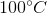.  What is the pressure at?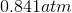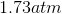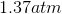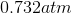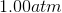Explanation:

The pressure of the flask atis.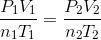Because the volume between the flasks and the moles in each flask are constant, we can cancel outand.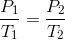At STP, conditions areand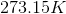.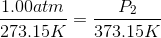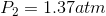### Example Question #1 : Gases

A 3.00 L container at 273 K is filled with 1.00 mol Cl2(g), which behaves non-ideally.

Using the van der Waals equation, calculate pressure exerted by the gas.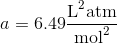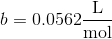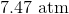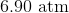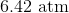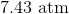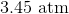Explanation:

Recall the van der Waals equation for non-ideal gases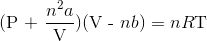Rewrite the equation using the known values and solve for P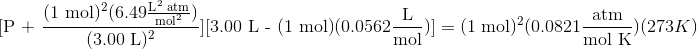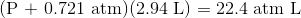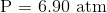### All College Chemistry Resources